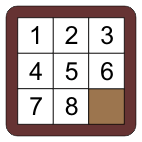2 views

I am solving the 8-puzzle. It is a problem which looks like this:Image courtesy of https://ece.uwaterloo.ca/~dwharder/aads/Algorithms/N_puzzles/ (you can also see there for a more detailed description of the 8-puzzle). The user can move a square adjacent to the blank into the blank. The task is to restore the arrangement as shown in the picture, starting from some arbitrary arrangement.

Now, of course, the state can be described as a permutation of 9 digits. In the case of the picture shown, the permutation is:

1 2 3 4 5 6 7 8 0

However, not all permutations are reachable from the shown configuration. Therefore, I have the following questions.

1. What is the number of permutations obtainable from the shown initial configuration by sliding tiles into the blank?

2. Call the answer to the above N. Now, I want a 1-1 mapping from integers from 1 to N to permutations. That is, I want to have a function that takes a permutation and returns an appropriate integer as well as a function that takes an integer and returns the permutation. The mapping has to be a bijection (i.e. an imperfect hash is not enough).

by (108k points)

1. There are exactly two equivalence classes of reachable configurations. The 8-puzzle has a property that exactly half (of all) permutations can be reached from any starting state.